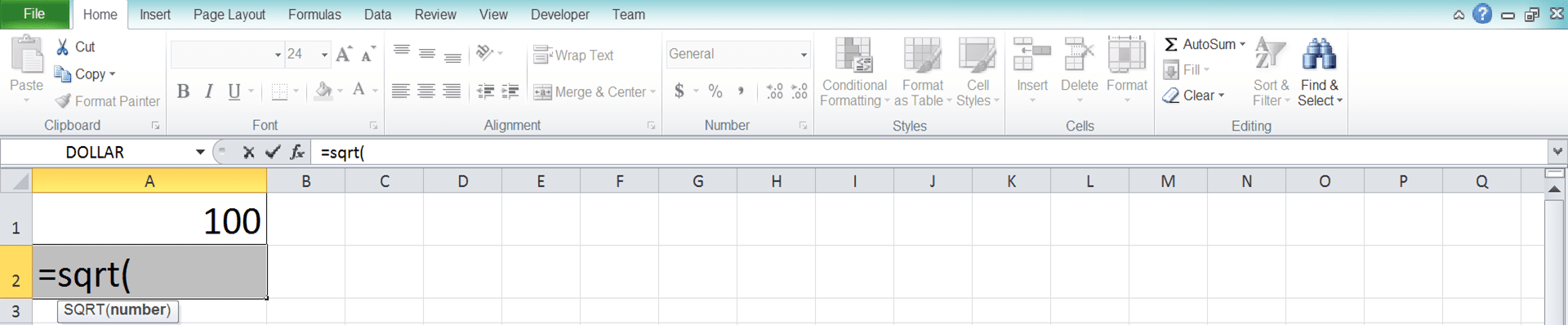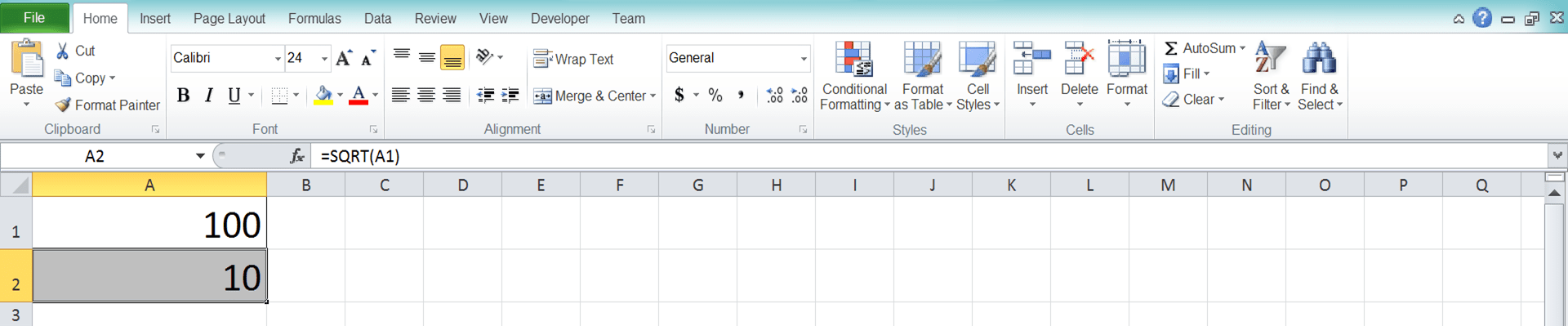How to Use Excel SQRT Function: Usability, Examples, and Writing Steps - Compute Expert

# How to Use Excel SQRT Function: Usability, Examples, and Writing Steps

Home >> Excel Tutorials from Compute Expert >> Excel Formulas List >> How to Use Excel SQRT Function: Usability, Examples, and Writing Steps

In this tutorial, we will discuss all things about the SQRT excel function. This function can help you if you want to calculate the square root (second power level of the root) of a number in excel.

Disclaimer: This post may contain affiliate links from which we earn commission from qualifying purchases/actions at no additional cost for you. Learn more

## SQRT Usefulness

SQRT can be used to get the square root calculation result (second power level of the root) from a number in excel.

## SQRT Result

The result you get from SQRT is the square root calculation result of your number.

## Excel Version in Which SQRT Can Start to Be Used

SQRT can be used since excel 2003.

## The Way to Write It and Its Input

Generally, SQRT writing in excel can be illustrated as follows.

=SQRT(number)

The only input this formula needs is number, which is the number you want to square root.

## Example of Usage and Result

The following will give and explain the SQRT usage example in excel and the result you can get from it.As you can see in the example, SQRT can calculate the square root of a number easily. You just need to input the number you want to square root into SQRT and you will immediately get the result.

On an important note, you must ensure the number you input isn’t negative so SQRT won’t produce an error. If you aren’t sure whether your number is positive or not, learn from the SQRT ABS combination part of this tutorial.

## Writing Steps

The following will explain the steps to write SQRT in excel in detail. Each step will be explained with a screenshot to make you understand it easier.

1. Type an equal sign ( = ) in the cell where you want to put the square root result of your number2. Type SQRT (can be with large and small letters) and an open bracket sign after =3. Input the number you want to square root or the cell coordinate where the number is, after the open bracket sign4. Type a close bracket sign5. Press Enter
6. The process is done!## The Way to Make Sure Your Number is Positive So SQRT Won’t Produce a #NUM Error: SQRT ABS

One important thing you must make sure of when using SQRT is the number you input shouldn’t be negative. This is because a negative number can make SQRT produces a #NUM error (The square of a number is never negative and, because of that, its square root calculation cannot be done. The reason is a negative number doesn’t have a square root calculation result).

So, what if you aren’t sure your SQRT input is a positive number? Probably, you get the number from a specific calculation process that you do before. Or your number is a negative one but you want to turn it into positive so you can square root it?

To make sure a number is positive easily, you can use ABS. This formula can change a negative number into a positive one. If the number is already positive, it won’t do anything to that number.

Generally, the ABS formula writing in excel itself is as follows:

=ABS(number)

If it is combined with SQRT to ensure your number is positive before it is square rooted, the writing becomes this.

=SQRT(ABS(number))

Pretty easy, isn’t it? By inputting your number into ABS before processed by SQRT, you won’t get an error because of a negative number input.

To make the ABS and SQRT combination explanation much clearer, here is an example that shows its usage impact in excel.

SQRT a negative number without ABS:SQRT a negative number with ABS:So, how is it? Have you seen the impact that ABS gives to the SQRT result, especially if its input is a negative number? ABS will help you convert any number you give as the SQRT input into a positive number. This is like what you can see in the “before” and “after” examples above.

By using ABS, then you make sure the number you input in SQRT is positive. The number which is already positive won’t be changed by ABS.

If you don’t use ABS when the input is negative, you will get a #NUM error as in the “before” example.

## Calculation of Other Nth Roots

What if what you need is a root calculation with the power level besides 2 (not a square root)? Unfortunately, you cannot use SQRT for that. However, you can write the root formula in your cell manually or use POWER.

Generally, the manual writing of a root formula in excel is as follows.

=number_you_want_to_root^(1/the_power_level_of_the_root)

On the other hand, the POWER formula writing for a root calculation is as follows.

=POWER(number_you_want_to_root,1/the_power_level_of_the_root)

In those writings above, you need the inputs of the number you want to root and the root’s power level. The manual root formula writing in excel can be done by using the caret symbol (^) help.

The ^ symbol and the POWER formula themselves are meant to calculate power. However, because root is the opposite of power, then those two methods can be used to calculate root too.

To use the ^ symbol and POWER for the root calculation, we must input “1/“ before inputting the power level. This is important to reverse the power calculation process that they do into a root one.

As for both implementations’ example to do the root calculation, see the following screenshot.In the example, we can see how to write a root formula manually, the POWER usage, and also their results. You just need to input the number you want to root and the root’s power level as explained before.

These two methods can also be used to square root a number if you want (input 2 as the root’s power level).

## Exercise

After you have learned the tutorial of SQRT above, let’s do an exercise to deepen your understanding.

### Questions

1. What is the SQRT result for the first number?
2. What is the SQRT result for the second one?
3. What is the SQRT result for the third?

SQRT is one of the fastest ways to square root a number in excel. If the calculation is what you need, then using this formula is recommended.

Related tutorials you should learn:

Get updated excel info from Compute Expert by registering your email. It's free!